### Home > PC > Chapter 4 > Lesson 4.2.1 > Problem4-71

4-71.
1. Find all values of θ from [0, 2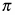] where the following equations are true. Sketching a picture of the unit circle may help. Homework Help ✎

1. sin θ =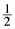2. cos θ = −3. sin θ =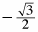4. cos θ = 0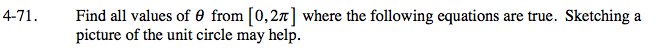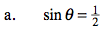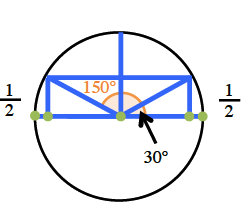$30\degree\text{ or }\frac{\pi}{6}$

$150\degree\text{ or }\frac{5\pi}{6}$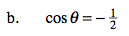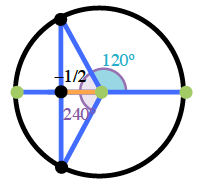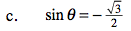What special angle(s) are involved?
What does the negative sign mean?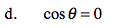These angles are coterminal with the y-axis.